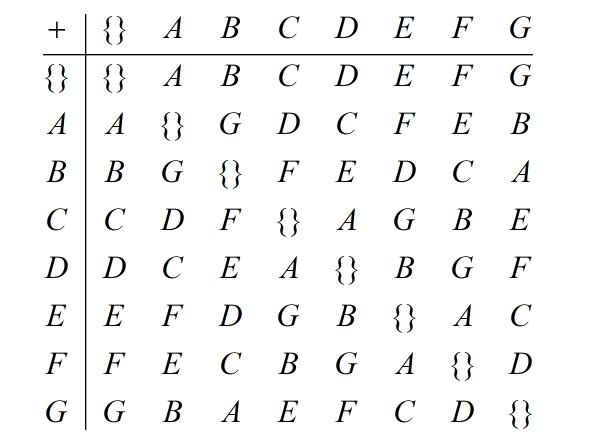None:
Polyps:
Strongs:RevelationThe Peculiar Structure Of The Viols Returning back to th point where one of the four beasts give the seven viols of wrath to the seven angels we have the sum of rows: [a,c,e,g] + a = [0,b,d,f] = 1 [b,f,a,e] + a = [0,c,d,g] * [d,g,b,a] + a = [0,c,e,f] * [c,e,d,b] + a = [b,d,e,c] [f,a,c,d] + a = [0,g,b,e] * [g,b,f,c] + a = [f,c,g,b] [e,d,g,f] + a = [d,e,f,g] Now we have [b,d,e,c], [f,c,g,b], [d,e,f,g] form a K4 group under symmetric difference. However under symmetric difference any two elements form a third from the set in the rightmost column. (We have closure) We also have (x + y) + y = x and also (x+y)+z=x+(y+z) (inverses and associativity) The empty set fulfils the role of the additive identity. (So we have a group.) Thus [a,c,e,g] + a = [0,b,d,f] = 1 = A [b,f,a,e] + a = [0,c,d,g] = B [d,g,b,a] + a = [0,c,e,f] = D [c,e,d,b] + a = [b,d,e,c] = C [f,a,c,d] + a = [0,g,b,e] = F [g,b,f,c] + a = [f,c,g,b] = G [e,d,g,f] + a = [d,e,f,g] = E fulfils the table of addition as below.The viols of wrath not only give an octal reversal, they add to the cosets of the "trumps" (our reference octal) an operation after the manner of the octal yet with the three groups in the K4 form (a,b,c), (a,d,e), (a,f,g) excluded. What is the importance of this? Clearly if we equate the K4 form that spans the octal to Christ, then there is here an octal outside of or aside from Christ that remains for the ungodly. This structure itself appears to be the inheritance of those doomed to the lake of fire - possibly becoming the lake itself. Given our original seven cycle (a,b,d,c,f,g,e) the four possible static subgroups are present in the above octal of "wrath" yet curiously not the four possible antichrist bows. We would expect the lake of fire to contain the bows and provide a cage for the dialectic. What octal do we need to start from to provide this? Clearly the wormwood octal we saw previously. Then we would have for a =1 [d,e,f,g] + a = [0,a,b,c]c = [d,e,f,g] [b,c,f,g] + a = [0,a,d,e]c = [b,c,f,g] [b,c,d,e] + a = [0,a,f,g]c = [b,c,d,e] [a,b,e,g] + a = [0,c,d,f] [a,b,d,f] + a = [0,c,e,g] [a,c,e,f] + a = [0,b,d,g] [a,c,d,g] + a = [0,b,e,f] So our starting octal is the wormwood set from earlier. So, the wrath as poured out begins to be poured out on the earth of the "sun octal" , and in seven stages (viols) transforms towards this wormwood octal that finally becomes the last cage for antichrist and every "foul and detestable spirit". In isomorphism the octals align as one field GF(8). In that case all that remains is the K4 form and the two sets numbering 666 to left and right. This "wrath" octal is the one that is found outside of the K4 form. It must then be the only refuge for the beast and antichrist - the lake of fire perhaps? Since the viols are poured out on the sea there is no preference to which is the reference octal; However if there is one "sun octal" it must be somehow excluded from becoming the "lake of fire". This "sun octal" opposes "that singular one of Euphrates - (one of the four rivers generated by the seven cycles on the wormwood octal.) and vice versa. Then each octal opposes its wormwood counterpart with which its "water" is exchanged for "blood". The octals then, with their cosets now forming the closure of the four bows under symmetric difference (A v B) are a closed set (a cage) for the antichrist using the dialectic - it is the only fleeting refuge from the winepress of the wrath of God, and is strictly outside of Christ. We are not shifting unity but static groups in the winepress here (acting as on trumpets) - in effect we found a cage (as the image) not in the earth but within the sea. The winepress forces antichrist into the lake of fire, whereas the woman "which sits on many waters" is a cage in the sea now become the lake of fire, that contains every nation except spiritual Israel, the elect in Christ. Continue To Next Page Return To Section Start Return To Previous Page'